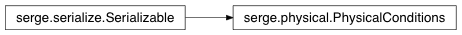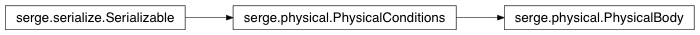# Physical¶

Contents

## PhysicalConditions¶class `serge.physical.``PhysicalConditions`(mass=0.0, radius=0.0, velocity=(0.0, 0.0), force=(0.0, 0.0), width=0.0, height=0.0, fixed=False, friction=0.1, elasticity=1.0, group=0, layers=-1, update_angle=False, visual_size=False)[source]

Represents physical parameters of an object

This includes the mass, velocity, force applied, acceleration and the physical dimensions.

`init`()[source]

Initialize from serialized form

`setGeometry`(radius=None, width=None, height=None)[source]

Set the geometry

You must specify either the radius or the width and height

`updateFrom`(physical_conditions)[source]

Update the properties and our physics object

## PhysicalBody¶class `serge.physical.``PhysicalBody`(mass, **kw)[source]

Physical conditions for an infinitesimal object

The object has no dimensions (shape) but still has mass etc.